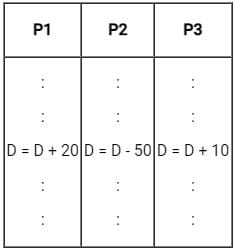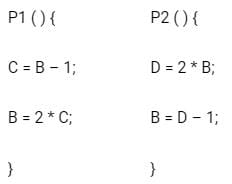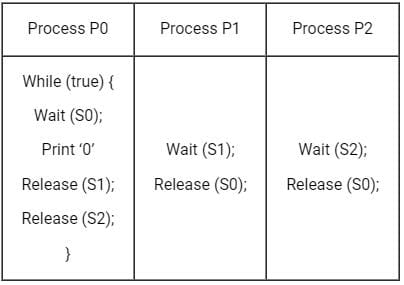Test: Concurrency & Synchronization

# Test: Concurrency & Synchronization

Test Description

## 10 Questions MCQ Test Operating System | Test: Concurrency & Synchronization

Test: Concurrency & Synchronization for Computer Science Engineering (CSE) 2023 is part of Operating System preparation. The Test: Concurrency & Synchronization questions and answers have been prepared according to the Computer Science Engineering (CSE) exam syllabus.The Test: Concurrency & Synchronization MCQs are made for Computer Science Engineering (CSE) 2023 Exam. Find important definitions, questions, notes, meanings, examples, exercises, MCQs and online tests for Test: Concurrency & Synchronization below.
Solutions of Test: Concurrency & Synchronization questions in English are available as part of our Operating System for Computer Science Engineering (CSE) & Test: Concurrency & Synchronization solutions in Hindi for Operating System course. Download more important topics, notes, lectures and mock test series for Computer Science Engineering (CSE) Exam by signing up for free. Attempt Test: Concurrency & Synchronization | 10 questions in 30 minutes | Mock test for Computer Science Engineering (CSE) preparation | Free important questions MCQ to study Operating System for Computer Science Engineering (CSE) Exam | Download free PDF with solutions
 1 Crore+ students have signed up on EduRev. Have you?
Test: Concurrency & Synchronization - Question 1

### Implementation of ________ may waste CPU cycles.

Detailed Solution for Test: Concurrency & Synchronization - Question 1

Spinlock: It is also called Busy Waiting. It is a process synchronization technique that makes the process (task) wait for a particular operation to complete or check for a condition to satisfy before proceeding with its execution.

• Spinlock wastes CPU cycles. The processes are busy checking the instructions and waiting to enter the critical section is called busy waiting.

Sleep and Wake: It is a very simple concept where a process that wants to enter into the critical section but the critical section is busy then the process will go to sleep. It will be woken by the other process which is currently in the critical section so that the sleeping process can wake up and enter into the critical section. This process is done to prevent the wastage of CPU cycles.

Test: Concurrency & Synchronization - Question 2

### Busy Waiting in process synchronisation is a term used when a process is in critical section and other processes are waiting to enter critical section. Busy Waiting is also called ___________

Detailed Solution for Test: Concurrency & Synchronization - Question 2

Bounded Waiting: This is a condition that needs to be fulfilled to achieve synchronisation.

• It says that no process has to wait forever to enter into a critical section.
• There should be a specific time after which it should get a chance to enter into the critical section.
• If bounded waiting is not fulfilled, it can suffer from starvation.

Busy processing: There is no such thing as busy processing.
Spin Lock: Also called Busy Waiting.

• ​When a process is in the critical section and other processes are waiting to enter the critical section then it is called spinlock.
*Answer can only contain numeric values
Test: Concurrency & Synchronization - Question 3

### Consider three concurrent processes P1, P2 and P3 as shown below, which access a shared variable D that has been initialized to 100.The processes are executed on a uniprocessor system running a time-shared operating system. If the minimum and maximum possible values of D after the three processes have completed execution are X and Y respectively, then the value of Y - X is __________.

Detailed Solution for Test: Concurrency & Synchronization - Question 3

Maximum value (Y):

• Process P1 reads the initial value 100 during execution P1 will update the value of D to (100 + 20 = 120) and writes it to memory.
• P2 and P3 read the value 120 from the memory.
• P2 will get executed before P1 and write the value (120 - 50 = 70) to memory. Since P3 is holding D = 120 after its execution D will be equal to (120 + 10 = 130) ∴ Y = 130

Minimum value (X):

• P1, P2 and P3 read the value 100 from the memory
• P1 will execute first, after its execution it will update (D = 100 + 20 = 120)
• After P1, P3 will execute and it will update the value to (D = 100 + 10 = 110)
• Finally, P2 will be execute and D will be equal to 100 - 50 = 50 ∴ X = 50
• Y - X = 130 - 50 = 80
Test: Concurrency & Synchronization - Question 4

At a particular time of computation the value of a counting semaphore is 7. Then 20 P operations and 15 V operation were completed on this semaphore. The resulting value of the semaphore is

Detailed Solution for Test: Concurrency & Synchronization - Question 4

Concepts:
V(S): Signal operation will increment the semaphore variable, that is, S++.
P(S): Wait operation will decrement the semaphore variable., that is, S--.

Data:
Initial counting semaphore = I = 7
Wait operation = 20 P
Signal operation = 15 V
Final counting semaphore = F

Formula:
F = I + 15V + 20P

Calculation:
F = 7 + 15(+1) + 20(-1)
∴ F = 2
the resulting value of the semaphore is 2

Test: Concurrency & Synchronization - Question 5

Which of the following is not an optimization criterion in the design of a CPU scheduling algorithm?

Detailed Solution for Test: Concurrency & Synchronization - Question 5

Maximum CPU utilization, maximum throughput, minimum turnaround time, and Minimum waiting time are the optimization criterion in the design of a CPU scheduling algorithm

*Answer can only contain numeric values
Test: Concurrency & Synchronization - Question 6

The following two functions P1 and P2 that share a variable B with an initial value of 2 execute concurrently.The number of distinct values that B can possibly take after the execution is __________.

Detailed Solution for Test: Concurrency & Synchronization - Question 6

Initial value of B is 2 //value stored in main memory
Value of B = 4
First execute P2()
P2 ( ) {
D = 2 * B;   // 2 × 2 = 4
B = D – 1; // B = 4 – 1 = 3
}
write the value of B = 3 to memory
execute P1()
P1 ( ) {
C = B – 1; // C = 3 – 1 = 2
B = 2 * C; // B = 2 × 2 = 4
}
write the value of B = 4 is memory.
Distinct value of B is 4.
Value of B =3
First Execute P1()
P1 ( ) {
C = B – 1; // C = 2 – 1 = 1
B = 2 * C; // B = 2 × 1 =2
}
write the value of B = 2 to memory
then execute P2()
P2 ( ) {
D = 2 * B;   // 2 × 2 = 4
B = D – 1; // B = 4 – 1 = 3
}
write the value of B = 3 to memory
One of the distinct values of B is 3.
Value of B = 2
First execute P2()
P2 ( ) {
D = 2 * B;   // 2 × 2 = 4
B = D – 1; // B = 4 – 1 = 3
}
//don’t write to memory
execute P1()  // with initial value of B = 2
P1 ( ) {
C = B – 1; // C = 2 – 1 = 1
B = 2 * C; // B = 2 × 1 = 2
}
write the value B = 3 by P2() in memory.
overwrite the value written by P2() as B = 2 by P1() in memory.
One of the distinct values of B is 2.
Therefore, the distinct values of B are 2, 3 and 4.
The number of distinct values that B can possibly take after the execution is 3.

Test: Concurrency & Synchronization - Question 7

Mutex can also be referred to as ___________

Detailed Solution for Test: Concurrency & Synchronization - Question 7

Semaphores: It is a non-negative integer value used by various processes mutually exclusive to achieve synchronization. A semaphore S can be accessed by only two operations which are atomic and mutually exclusive in nature - Wait (P) and Signal (V). There are two types of semaphores:

• Counting Semaphore: In this, the value of the semaphore is from an unrestricted integer domain, that is, it can have an integer value as high as we want.
• Binary Semaphore: In this, the value can range only between 0 and 1.

Monitor: It is a high-level construct implemented using a programming language that packages the data and procedures to modify the data. Only one processor is allowed in the monitor at a time.
Mutex: It is a locking mechanism used to synchronize access to a resource. Only one task can acquire the mutex, hence, ownership is associated with mutex and only the owner can release the lock. This whole system can be implemented using a binary semaphore hence, it is referred to as a binary semaphore.

Test: Concurrency & Synchronization - Question 8

Consider a counting Semaphore variable 'S'. Following are the semaphore operations performed: 18P, 3V, 7V, 2P, 6V. What can be the largest initial value of S to keep one process in suspended list?

Detailed Solution for Test: Concurrency & Synchronization - Question 8

Semaphore: it is an integer variable which is used by different processes in a mutually exclusive manner so as to achieve synchronisation.
There are two types of Semaphores:

• Counting Semaphores: The integer values in this type of semaphore can range over more than 0s and 1s
• Binary Semaphores: The integer values in this type of semaphore can only be binary in nature, that is, 0s and 1s.

There are two types of operations that are atomic and mutually exclusive in nature:

• Wait (S) or P: If the semaphore value is greater than 0, decrement it. If it is less than 0, then wait for the semaphore to become greater than 0 and then decrement it.
• Signal (S) or V: Increment the value of the semaphore. If the semaphore is of binary type, and is already 1, then again replace the value by 1.

Calculations:

• Let the initial value of counting semaphore be 'x'.
• One process is in suspend list, which makes the final value to be -1.
• −1=x−18+3+7−2+6
• −1=x−20+16
• x=20−16−1
Test: Concurrency & Synchronization - Question 9

The following program consists of 3 concurrent processes and 3 binary semaphores. The semaphores are initialized as S0 = 1, S1 = 0, S2 = 0.How many times will process P0 print ‘0’?

Detailed Solution for Test: Concurrency & Synchronization - Question 9

Concept:
Binary semaphore which can take only two values 0 and 1 and ensure mutual exclusion.
A process is entered into the critical section only when the value of semaphore(s) = 1
Otherwise, it will wait until semaphore(s) >0

The semaphores are initialized as S0=1, S1=0, S2=0.
Because S0 =1 then P0 enter into the critical section and other processes will wait until either S1=1 or S2 =1
The minimum number of times 0 printed:

• S0 =1 then P0 enter into the critical section
• print '0'
• then release S1 and S2 means S1 =1 and s2 =1
• now either P1 or P2 can enter into the critical section
• if P1 enter into the critical section
• release S0
• then P2 enter into the critical section
• release S0
• P1 enter into the critical section
• print '0'

The minimum number of time 0 printed is twice when executing in this order (p0 -> p1 -> p2 -> p0)
The Maximum number of times 0 printed:

• S0 =1 then P0 enter into the critical section
• print '0'
• Then release S1 and S2 means S1 =1 and s2 =1
• Now either P1 or P2 can enter into the critical section
• If P1 enter into the critical section
• Release S0 means S0 =1
• S0 =1 then P0 enter into the critical section
• print '0'
• Then P2 enter into the critical section
• Release S0 means S0 =1
• S0 =1 then P0 enter into the critical section
• print '0'

Maximum no. of time 0 printed is thrice when execute in this order (p0 -> p1 -> p0 -> p2 -> p0)
So, At least twice will process P0 print ‘0’

Test: Concurrency & Synchronization - Question 10

Two atomic operations permissible on Semaphores are __________ and __________.

Detailed Solution for Test: Concurrency & Synchronization - Question 10

Semaphores are integer variables that are used to solve the critical section problem by using two atomic operations, wait and signal that are used for process synchronization.

Wait:
The wait operation decrements the value of its argument S if it is positive. If S is negative or zero, then no operation is performed.

wait(S)

{

while (S<=0)

S--;

}

Signal:

The signal operation increments the value of its argument S.

signal(S)

{

S++;

}

## Operating System

80 docs|33 tests
 Use Code STAYHOME200 and get INR 200 additional OFF Use Coupon Code
Information about Test: Concurrency & Synchronization Page
In this test you can find the Exam questions for Test: Concurrency & Synchronization solved & explained in the simplest way possible. Besides giving Questions and answers for Test: Concurrency & Synchronization, EduRev gives you an ample number of Online tests for practice

80 docs|33 tests